## DFHRS - Kosovo 2015

The DFHRS Database of Kosovo was computed in 2015 in the frame of Research visit of Dr. Perparim Ameti (2015) from University of Prishtina. The 6 months research work "On the definition of height elevation surfaces over an arbitrary selected area by means of DFHRS approach" has been realized in cooperation with Prof.dr. Reiner Jaeger at Institute fuer Angewandte Forschung/Hochschule Karlsruhe Technik und Wirtschaft (HSKA). The computations according to the DFHRS approach were based on the geopotential model EGM2008, EGG97, EGM96 and EIGEN05c. The whole area of the Kosovo has been used for further analysis and determination of the best solution of a height reference surface (HRS), by using DFHRS technique. Kosovo has an area of app. 11,000km² with an average altitude from 300  2500m above mean sea level. For the computation of height reference surface (geoid model), 30 points of 1st order GPS network has been chosen as target values of normal heights (Fig. 1). These points have three dimensional coordinates determined by GPS in ETRS89, as well as the height determined by precise leveling. The Kosovo 1st order network consists of 32 points, whereby two points have been neglected from computation because of the critical deviation in the height value. After establishment of the Kosovo geodetic datum in 2001, the 1st order network has been densified with about 450 points covering whole territory of Kosovo with an approximate distance of 10 to 15km between neighbor points. Currently, these points are serving as height reference surface, however their deviation in some areas exceed ±50cm and need to be considered.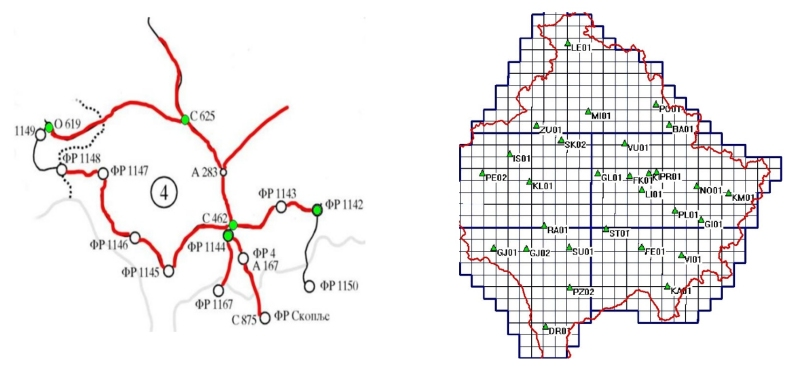Fig. 1 Precise leveling (Left) and GPS control network (Right) of Kosovo

The general computational principle of the DFHRS approach is to divide the whole area of a continuous HRS into an appropriate number of patches. For further calculations, sufficient points in both surfaces (at least 4 points) must be known. The patches are composed as part of the whole HRS and are divided into number of regular meshes (5km x 5km) as shown in the Figure 2.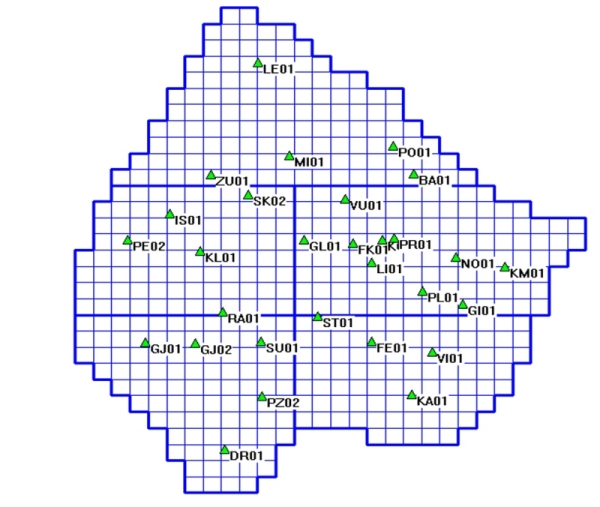Fig. No. 2 Definition of patches and mashes

Each patch contents a datum and related transformation parameters (d) and each mesh have HRS parameters (p). The DFHRS parameters (p) and the mesh information are stored in an appropriate file at data base of DFHRS software. In this approach the continuity condition has been considered, whereby, for the NFEM point in the boundary between two meshes should be depending for both meshes (C0-continuity) and the slope at the boundary of the meshes should represent the whole area (C1-continuity). In order to reduce the effect of medium or long-wave length, systematic shape deflections in the observations N and in the components of the deflection of vertical derived from GPM or other sources, the DFHRS concept predicts subdivision of any geoid model into a number of so called geoid patches, see Figure 2. The DFHRS concept foresees the continuity between neighboring meshes in order to predict a uniform height reference surface which is consistent over whole area.

### Geoid Analyses and Results

For the computation of Kosovo geoid model, 30 points of Kosovo 1st order network has been used. These points have known ellipsoidal coordinates in the ETR89 as well as normal heights from precise leveling network of Kosovo. The physical components of the gravity are derived from global geopotential models (EGM2008, EGM96, EGG97 and EIGEN05C). For the quality control of the calculated surface, selected number of the 1st order network has been used. Additional test has been done in order to define the necessity of the determination of the vertical datum by taking into account reference surface modeling, for this purpose, a DEM was built by more than 450 points of geodetic control networks and then compared with determined geoid model. The whole area was divided into a grid of regular meshes of size 5km x 5km and for the deviation of possible systematic errors, 5 patches with an apriority accuracy of 1cm, were used to avoid long and medium wave components in the different regional areas. Table No. 1, shows the comparison of geoidal heights with the actual heights determined by GPS/leveling. Analyzing the table we can conclude that the GPM EGM2008 (Pavlis, 2008) and EGG97 (Denker and Torge, 1998) provided best results in comparison to EGM96 (Leomoine et al. 1998) and EIGEN05C (Förste et al. 2007). Interpreting the Table 1, results have provided an equal standard deviation when using the EGM2008 and EGG97 potential model (Std=0,03cm).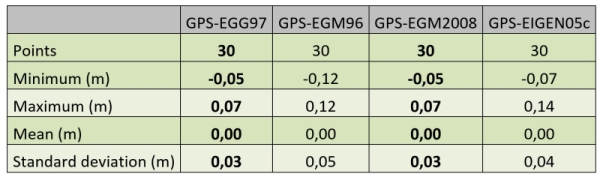Table No. 1 Geoid height residuals to GPS/Leveling points

Table 1 presents a statistical summary of the residual geoid height values obtained by using different GPM in the DFHRS approach. The EGM2008 and EGG97 models offer very good accuracy and we can clearly identify that only 5 of 30 points exceed the accuracy value of 0.04cm. The rest of 25 points have been determined with an accuracy of better than 4cm and they cover the majority of the whole area.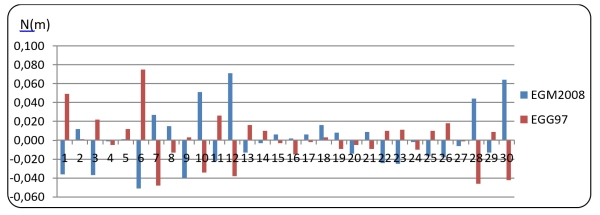Chart No. 1 Residual geoidal heights N

Chart 2, shows residual values of geoid height computed by using all geopotential models (EGM2008. EGG97, EGM96, EIGEN05C). A prevail of large deviation of residual values when using EGM96 or EIGEN05C is evident. In other side the results coming from the EGM2008 and EGG97 lies close to each other.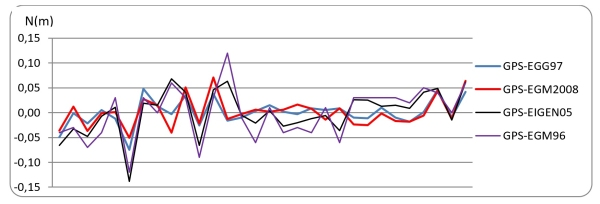Chart No. 2 Geoid height residuals to GPS/Leveling points

The difference between the geoid model calculated from EGM2008 and the geoid model calculated from EGG97 is smaller than ±3cm. The difference model doesnt show any coherent reason for this deviation (See Figure 3 and Chart 1).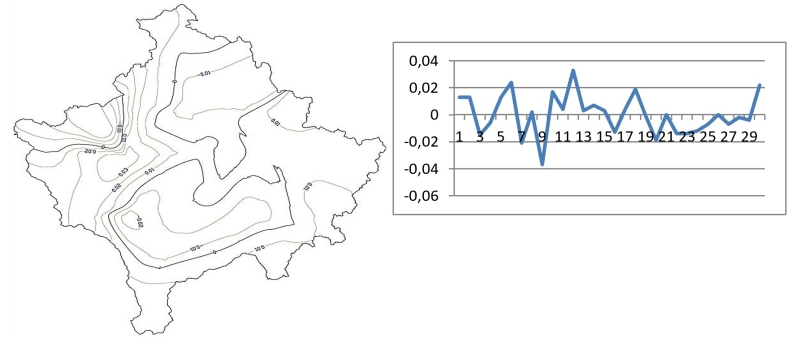Fig. 3 Difference model calculated from EGM2008 and EGG97

This deviation may possibly be reason by the lack of the terrestrial data used during the determination of both above mentioned global potential models and the representation of the gravity field contains only long-wave characteristics. The difference itself of the global potential model EGM2008 and EGG97 for the territory of Kosovo has been extracted and analyzed; calculation tests yielded a difference of range appr. 1m.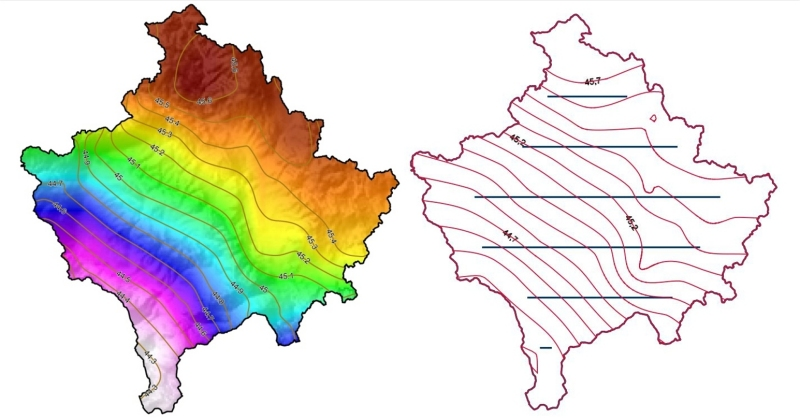Fig. 4 Final Kosovo geoid model determined by DFHRS concept

The EGM2008 has been selected for the final solution of Kosovo geoid model (Figure 4), even it has similar statistical accuracy with EGG97. Analyses have acknowledged that EGM2008 fits better with the topographic dispersion over the territory of Kosovo. The final HRS as such can be considered as accurate enough and can be imposed as the height reference model in the Kosovo positioning online system (KOPOS).